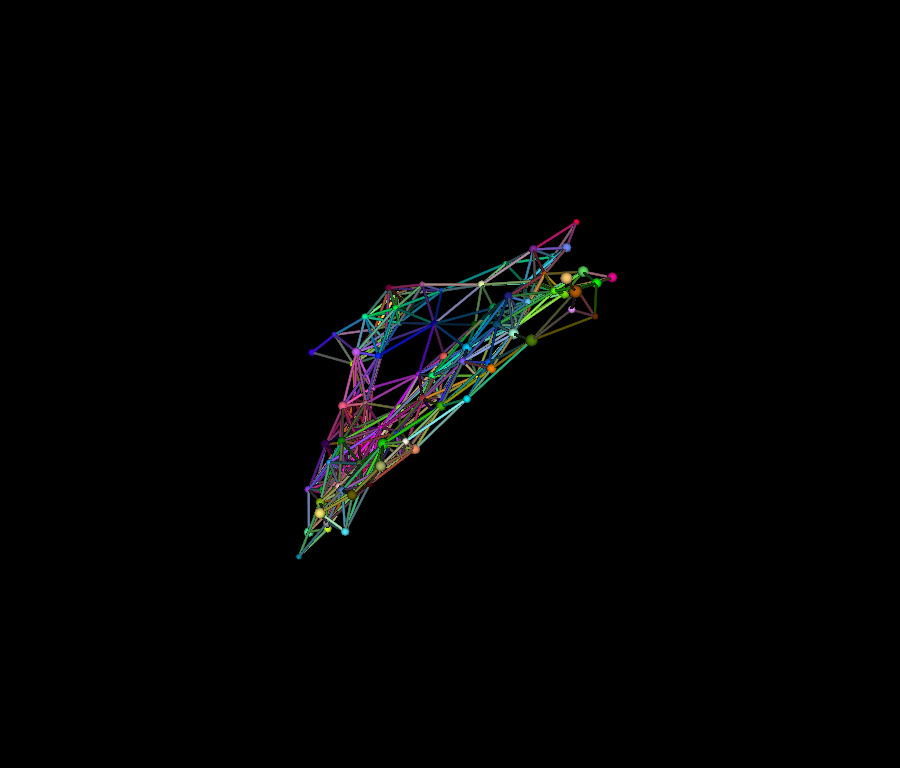# Visualize Networks (Animated version)#

The goal of this demo is to show how to visualize a complex network and use an force directed algorithm to layout the network. A simpler animation of the network made by adding some random displacements to nodes positions is also demoed.

First, let’s import some useful functions

```import math
from os.path import join as pjoin

import numpy as np

from fury import actor
from fury import colormap as cmap
from fury import window
from fury.utils import compute_bounds, update_actor, vertices_from_actor
```

This demo has two modes. Use mode = 0 to visualize a randomly generated geographic network by iterating it using a force-directed layout heuristic.

Use mode = 1 to visualize a large network being animated with random displacements

```mode = 0
```

```if mode == 1:
from fury.data.fetcher import fetch_viz_wiki_nw

files, folder = fetch_viz_wiki_nw()
categories_file, edges_file, positions_file = sorted(files.keys())
```

We read our datasets (mode 1)

```if mode == 1:
vertices_count = len(positions)
```

Generate a geographic random network, requires networkx package (mode 0)

```if mode == 0:
import networkx as nx

vertices_count = 100
view_size = 100
network = nx.random_geometric_graph(vertices_count, 0.2)
positions = view_size * np.random.random((vertices_count, 3)) - view_size / 2.0
categories = np.arange(0, vertices_count)
edges = np.array(network.edges())
positions = view_size * np.random.random((vertices_count, 3)) - view_size / 2.0
```

We attribute a color to each category of our dataset which correspond to our nodes colors.

```category2index = {category: i for i, category in enumerate(np.unique(categories))}

index2category = np.unique(categories)

category_colors = cmap.distinguishable_colormap(nb_colors=len(index2category))

colors = np.array(
[category_colors[category2index[category]] for category in categories]
)
```

We define our node size

```radii = 1 + np.random.rand(len(positions))
```

Let’s create our edges now. They will indicate a citation between two nodes. The colors of each edge are interpolated between the two endpoints.

```edges_colors = []
for source, target in edges:
edges_colors.append(np.array([colors[source], colors[target]]))

edges_colors = np.average(np.array(edges_colors), axis=1)
```

Our data preparation is ready, it is time to visualize them all. We start to build 2 actors that we represent our data : sphere_actor for the nodes and lines_actor for the edges.

```sphere_actor = actor.sphere(
)

lines_actor = actor.line(
np.zeros((len(edges), 2, 3)),
colors=edges_colors,
lod=False,
fake_tube=True,
linewidth=3,
)
```

Defining timer callback and layout iterator

```def new_layout_timer(
showm,
edges_list,
vertices_count,
max_iterations=1000,
vertex_initial_positions=None,
):
view_size = 500
viscosity = 0.10
alpha = 0.5
a = 0.0005
b = 1.0
deltaT = 1.0

sphere_geometry = np.array(vertices_from_actor(sphere_actor))
geometry_length = sphere_geometry.shape / vertices_count

if vertex_initial_positions is not None:
pos = np.array(vertex_initial_positions)
else:
pos = view_size * np.random.random((vertices_count, 3)) - view_size / 2.0

velocities = np.zeros((vertices_count, 3))

def iterate(iterationCount):
nonlocal pos, velocities
for _ in range(iterationCount):
forces = np.zeros((vertices_count, 3))
# repulstive forces
for vertex1 in range(vertices_count):
for vertex2 in range(vertex1):
x1, y1, z1 = pos[vertex1]
x2, y2, z2 = pos[vertex2]
distance = (
math.sqrt(
(x2 - x1) * (x2 - x1)
+ (y2 - y1) * (y2 - y1)
+ (z2 - z1) * (z2 - z1)
)
+ alpha
)
rx = (x2 - x1) / distance
ry = (y2 - y1) / distance
rz = (z2 - z1) / distance
Fx = -b * rx / distance / distance
Fy = -b * ry / distance / distance
Fz = -b * rz / distance / distance
forces[vertex1] += np.array([Fx, Fy, Fz])
forces[vertex2] -= np.array([Fx, Fy, Fz])
# attractive forces
for vFrom, vTo in edges_list:
if vFrom == vTo:
continue
x1, y1, z1 = pos[vFrom]
x2, y2, z2 = pos[vTo]
distance = math.sqrt(
(x2 - x1) * (x2 - x1)
+ (y2 - y1) * (y2 - y1)
+ (z2 - z1) * (z2 - z1)
)
Rx = x2 - x1
Ry = y2 - y1
Rz = z2 - z1
Fx = a * Rx * distance
Fy = a * Ry * distance
Fz = a * Rz * distance
forces[vFrom] += np.array([Fx, Fy, Fz])
forces[vTo] -= np.array([Fx, Fy, Fz])
velocities += forces * deltaT
velocities *= 1.0 - viscosity
pos += velocities * deltaT
pos[:, 0] -= np.mean(pos[:, 0])
pos[:, 1] -= np.mean(pos[:, 1])
pos[:, 2] -= np.mean(pos[:, 2])

counter = 0

def _timer(_obj, _event):
nonlocal counter, pos
counter += 1
if mode == 0:
iterate(1)
else:
pos[:] += (np.random.random(pos.shape) - 0.5) * 1.5
spheres_positions = vertices_from_actor(sphere_actor)
spheres_positions[:] = sphere_geometry + np.repeat(pos, geometry_length, axis=0)

edges_positions = vertices_from_actor(lines_actor)
edges_positions[::2] = pos[edges_list[:, 0]]
edges_positions[1::2] = pos[edges_list[:, 1]]

update_actor(lines_actor)
compute_bounds(lines_actor)

update_actor(sphere_actor)
compute_bounds(lines_actor)
showm.scene.reset_clipping_range()
showm.render()

if counter >= max_iterations:
showm.exit()

return _timer
```

All actors need to be added in a scene, so we build one and add our lines_actor and sphere_actor.

```scene = window.Scene()

camera = scene.camera()

```

The final step! Visualize the result of our creation! Also, we need to move the camera a little bit farther from the network. you can increase the parameter max_iteractions of the timer callback to let the animation run for more time.

```showm = window.ShowManager(
scene, reset_camera=False, size=(900, 768), order_transparent=True, multi_samples=8
)

scene.set_camera(position=(0, 0, -300))

timer_callback = new_layout_timer(
showm, edges, vertices_count, max_iterations=200, vertex_initial_positions=positions
)

# Run every 16 milliseconds